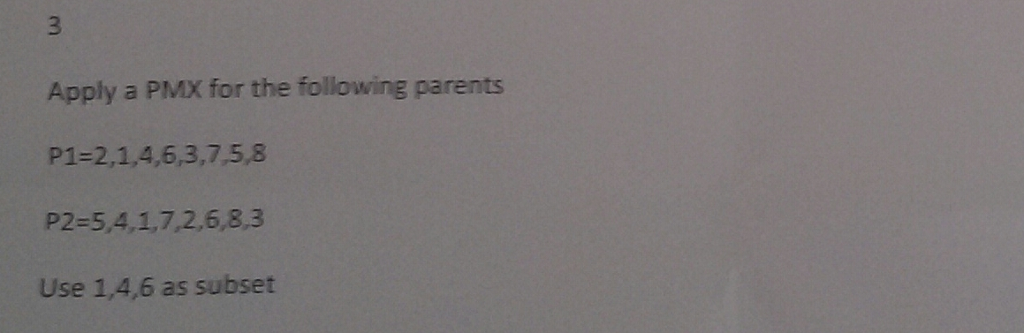# Answered! Apply a PVX for the following parents P_1 = 2, 1, 4, 6, 3, 7, 5, 8 P_2 = 5, 4, 1, 7, 2, 6, 8, 3 Use 1, 4, 6 as subset…Apply a PVX for the following parents P_1 = 2, 1, 4, 6, 3, 7, 5, 8 P_2 = 5, 4, 1, 7, 2, 6, 8, 3 Use 1, 4, 6 as subset

P1= 2,1,4,6,3,7,5,8

P2= 5,4,1,7,2,6,8,3
Subset = 1,4,6

Don't use plagiarized sources. Get Your Custom Essay on
Answered! Apply a PVX for the following parents P_1 = 2, 1, 4, 6, 3, 7, 5, 8 P_2 = 5, 4, 1, 7, 2, 6, 8, 3 Use 1, 4, 6 as subset…
GET AN ESSAY WRITTEN FOR YOU FROM AS LOW AS \$13/PAGE

Step1: swap segment

O1=(2 | 1 4 6 | 3 7 5 8)
O2=(5 | 4 1 7 | 2 6 8 3)
Now map between the subset

O1= (X | 4 1 7 | X X X X)
O2= (X | 1 4 6 | X X X X)
This defines mapping between 4&1, 1&4, 7&6

Step 2: fill the cities from other parents if no conflict occurs
O1= (2 | 4 1 7 | 3 X 5 8)
O2= (5 | 1 4 6 | 2 X 8 3)

Step 3: Use mappings for conflicted positions

O1= (2 | 4 1 7 | 3 6 5 8)
O2= (5 | 1 4 6 | 2 7 8 3)